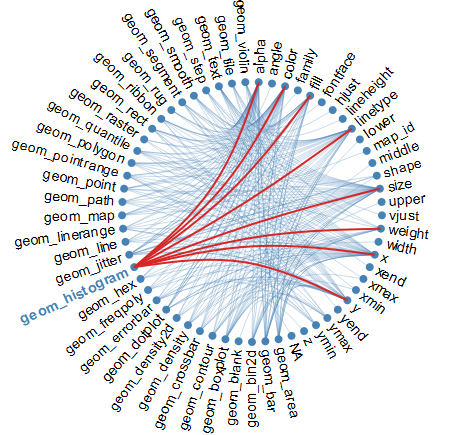Chord graph of geoms and aes parameters

# Mapping ggplot geoms and aesthetic parameters

The sheer variety of geom_* in ggplot2 is overwhelming and astounding, especially with the control over the many aesthetics such as shape and color. For example, the ggplot2 cheat sheet is packed (link).

I frequently forget which aes parameters exist for each geom_* and need a quick look at the help pages. Using the information from the cheatsheet, I was able to quickly summarize the aes parameters available for each geom_* (link).

And with the help of igraph and edgebundleR, a htmlwidget based on the D3 Javascript library, I created an interactive network visualizing the connection between geoms and aes parameters.

library(igraph)
library(edgebundleR)
library(dplyr)
library(purrr)
suppressPackageStartupMessages(library(widgetframe))

split_up <- function(x){
params <- unlist(strsplit(x, ","))
return(data.frame("from" = params, "to" = params[2:length(params)]))
}

relationship <- map_df(parameters, split_up)
head(relationship)

Perhaps not surprisingly, alpha is the most common aes parameter, followed closely by color and size.

knitr::kable(head(sort(table(relationship$to), decreasing = T), n = 10)) Var1 Freq alpha 33 color 30 size 30 x 29 linetype 26 y 24 fill 20 weight 9 ymax 8 ymin 7 geom_boxplot has the greatest number of aes parameters which can be controlled. knitr::kable(head(sort(table(relationship$from), decreasing = T), n = 10))
Var1 Freq
geom_boxplot 13
geom_text 11
geom_bin2d 10
geom_dotplot 10
geom_rect 9
geom_contour 8
geom_crossbar 8
geom_density 8
geom_errorbar 8
geom_histogram 8

Hover above the geom_* name to highlight the links to the aes parameters.

frameWidget(edgebundle(graph_from_data_frame(relationship), tension = 0.45, cutoff = 0.1, width = NULL, fontsize = 14,
padding = 110, nodesize = c(5, 20), directed = FALSE), height = "600")
## Warning in graph_from_data_frame(relationship): In d' NA' elements were
## replaced with string "NA"